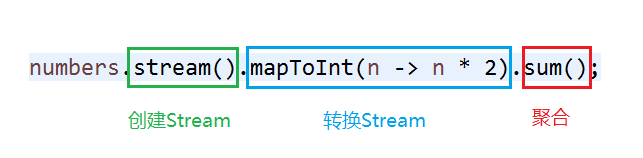2017/11/14 16:25

# 1.认识Stream

Java8对Stream的定义是这样的

A sequence of elements supporting sequential and parallel aggregate operations.

Stream的处理过程可分为三个部分，我们举个例子,对1,2,3三个数的平方求和。

``````List<Integer> numbers = Arrays.asList(1, 2, 3);
numbers.stream().mapToInt(n -> n * n).sum();
``````# 2.创建Stream

1. 通过集合类的相关方法
2. 通过Stream接口的静态工厂方法
3. 其他能产生流的类的方法

## 2.1 集合类的相关方法

Collection接口中定义了一个stream方法

``````default Stream<E> stream() {
return StreamSupport.stream(spliterator(), false);
}
``````

``````List<User> userList = // 数据库查询
long teenagerNum = userList.stream().filter(u -> u.getAge() < 20).count();
``````

``````int[] nums = {1, 2, 3, 2};
Arrays.stream(nums).distinct().forEach(System.out::println);
``````

distinct方法用来去重，即将一组整数去重后打印出来

## 2.2 Stream静态工厂方法

Stream接口中也提供多种静态方法来辅助我们构造Stream

1.of方法，非常好用的方法，接受可变参数

``````Stream<String> stringStream = Stream.of("1", "2", "3");
``````

``````String[] strs = {"1", "2", "3"};
Stream<String> stringStream = Stream.of(strs);
``````

2.generator方法，生成一个无限长度的Stream，需要一个自定义的Supplier类，比如创建一个随机数的Stream

``````Stream.generate(new Supplier<Long>() {
``````
``````    @Override
public Long get() {
return Math.random();
}
});
``````

``````Stream.generate(() -> Math.random());
Stream.generate(Math::random);
``````

3.iterate方法，同样生成无限长度的Stream，但它是对给定的种子(seed)反复调用用户指定函数来生成，其生成的元素可认为是：seed，f(seed)，f(f(seed))无限循环

``````Stream.iterate(1, i -> i + 1).limit(10).forEach(System.out::println);
``````

4.concat方法，将两个Stream组合成一个Stream

``````Stream<String> stream1 = Stream.of("1", "2", "3");
Stream<String> stream2 = Stream.of("4", "5");
Stream.concat(stream1, stream2);
``````

5.builder方法，使用追加的方式建立Stream然后消费

``````Stream.builder()
.build();
``````

## 2.3 其他方式

Java也在其他可能有Stream操作的类中增加了便捷的方法

``````// 文件读取
// 文件遍历
java.nio.file.Files.walk()
// 随机数
java.util.Random.ints()
// 正则匹配
Pattern.splitAsStream(java.lang.CharSequence)
``````

# 3.转换Stream

### 3.1 distinct

``````List list2 = new ArrayList(new HashSet(list));
``````

``````List list2 = list.stream().distinct().collect(Collectors.toList());
``````

### 3.2 filter

``````List<Integer> numbers = Arrays.asList(-1, 1, 0);
numbers.stream().filter(n -> n > 0).collect(Collectors.counting());
``````

### 3.3 map

``````Stream.of(1, 2, 3).map(n -> n + 1).collect(Collectors.toList());
``````

### 3.4 flatMap

``````[ [1, 2, 3], [4, 5],  ]
``````

``````[1, 2, 3, 4, 5, 6]
``````

``````Stream<List<Integer>> intStream = Stream.of(Arrays.asList(1, 2, 3),Arrays.asList(4,5),Arrays.asList(6));
intStream.flatMap(childList -> childList.stream()).forEach(System.out::println);
``````

### 3.5 peek

``````int sum = IntStream.range(1, 10).peek(System.out::println).sum();
System.out.println("sum:" + sum);
``````

### 3.6 limit

``````IntStream.range(1, 10).limit(5).forEach(System.out::println);
``````

### 3.7 skip

``````IntStream.range(1, 100).skip(10).limit(10).forEach(System.out::println);
``````

### 3.8 sorted

``````Stream.of(1, 3, 4 ,2).sorted().forEach(System.out::println);
``````

# 4.聚合Stream

### 4.1 collect

``````// 获取userId的List
List<Long> userIdList = users.stream().map(u -> u.getId()).collect(Collectors.toList());
// 获取userId的Set
Set<Long> userIdSet = users.stream().map(u -> u.getId()).collect(Collectors.toSet());
// 获取userId的Map
Map<Long, User> userIdMap = users.stream().collect(Collectors.toMap(u -> u.getId(), u -> u));
``````

``````Map<String, List<User>> userAddressMap = users.stream().collect(Collectors.groupingBy(User::getAddress));
``````

``````Map<Boolean, List<User>> userAgeMap = users.stream().collect(Collectors.partitioningBy(u -> u.getAge() > 20));
``````

Collectors中还有其他很便捷的方法，有兴趣的可以研究下。

### 4.2 reduce

``````Optional optional = users.stream().map(User::getAge).reduce((a1, a2) -> a1 > a2 ? a1 : a2);
``````

``````Integer maxAge = users.stream().map(User::getAge).reduce((a1, a2) -> a1 > a2 ? a1 : a2).get();
``````

``````Integer maxAge = users.stream().map(User::getAge).reduce(0 ,(a1, a2) -> a1 > a2 ? a1 : a2);
``````

• max(Comparator comp)
• min(Comparator comp)
• count()

``````users.stream().mapToInt(User::getAge).sum();
users.stream().mapToInt(User::getAge).average();
``````

### 4.3 搜索相关

Stream API还提供了几种快捷的搜索方法，支持在一组元素中的常用搜索。

• allMatch：所有元素都满足匹配条件
• anyMatch：任一元素满足匹配条件
• findFirst：返回Stream中的第一个元素
• findAny：返回Stream中的任意个元素
• noneMatch：所有元素都不满足匹配条件

### 4.4 遍历

``````users.stream().forEach(System.out::println);
``````

# 5.Stream API的优点

1. Stream转换操作是惰性化的(lazy),即多次转换操作在聚合操作时只需一次循环就能完成，并不会因为多次转换造成额外的循环开销。
2. 因为1的原因，Stream的数据源可以是无限的，它并不会像普通iterator一样需要把所有数据都加载到内存中。比如Stream的generate方法和limit方法相结合，可以从无限的数据源中操作自己想要的数据。
3. Stream可以并行化操作，它依赖于java7中引入的Fork/Join框架来拆分任务和加速处理过程。对于Collection子类，直接使用parallelStream方法即可开启并行化操作。不过并行化也是会有额外的开销的，因此要适当地使用。

# 6.Stream API用例

``````public class Stock {

// 主键id
Long id;
// 商品id
Long skuId;
// 供应商id
int supplierId;
// 状态(0:不可用,1:可用,2:任务中)
int status;
// 库存量
BigDecimal amount;
}
``````

### 6.1 从大集合中获取小集合

``````// 获取id的集合
List<Long> idList = stockList.stream().map(Stock::getId).collect(Collectors.toList());
// 获取skuid集合并去重
List<Long> skuIdList = stockList.stream().map(Stock::getSkuId).distinct().collect(Collectors.toList());
// 获取supplierId集合(supplierId的类型为int，返回List<Integer>,使用boxed方法装箱)
Set<Integer> supplierIdSet = stockList.stream().mapToInt(Stock::getSupplierId).boxed().collect(Collectors.toSet());
``````

### 6.2 分组与分片

``````// 按skuid分组
Map<Long, List<Stock>> skuIdStockMap = stockList.stream().collect(Collectors.groupingBy(Stock::getSkuId));
// 过滤supplierId=1然后按skuId分组
Map<Long, List<Stock>> filterSkuIdStockMap = stockList.stream().filter(s -> s.getSupplierId() == 1).collect(Collectors.groupingBy(Stock::getSkuId));
// 按状态分为不可用和其他两个分片
Map<Boolean, List<Stock>> partitionStockMap = stockList.stream().collect(Collectors.partitioningBy(s -> s.getStatus() == 0));
``````

### 6.3 计数与求和

``````// 统计skuId=1的记录数
long skuIdRecordNum = stockList.stream().filter(s -> s.getSkuId() == 1).count();
// 统计skuId=1的总库存量
BigDecimal skuIdAmountSum = stockList.stream().filter(s -> s.getSkuId() == 1).map(Stock::getAmount).reduce(BigDecimal.ZERO, BigDecimal::add);
``````

### 6.4 特定用法

``````// 多重分组并排序，先按supplierId分组，再按skuId分组，排序规则，先supplierId后skuId
Map<Integer, Map<Long, List<Stock>>> supplierSkuStockMap = stockList.stream().collect(Collectors.groupingBy(Stock::getSupplierId, TreeMap::new,
Collectors.groupingBy(Stock::getSkuId, TreeMap::new, Collectors.toList())));

// 多条件排序，先按supplierId正序排，再按skuId倒序排
// (非stream方法，而是集合的sort方法，直接改变原集合元素，使用Function参数)
stockList.sort(Comparator.comparing(Stock::getSupplierId)
.thenComparing(Stock::getSkuId, Comparator.reverseOrder()));
``````

http://ifeve.com/stream/ （强烈推荐，非常清晰的介绍）

http://www.infoq.com/cn/articles/java8-new-features-new-stream-api

https://www.ibm.com/developerworks/cn/java/j-lo-java8streamapi/

0
67 收藏

### 作者的其它热门文章2018/11/14 10:36

2017/12/06 20:30

2017/11/14 22:06

3 评论
67 收藏
0